## How to Calculate and Solve for Total Storage | Hydrology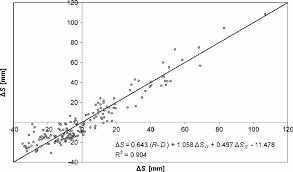The image above represents total storage.

To compute for total storage, three essential parameters are needed and these paramters are Storage co-efficient (k), Dimensionless constant (x) and Average outflow rate (O).

The formula for calculating total storage:

s = k[x + (1 – x)O]

Where:

s = Total Storage
k = Storage Co-efficient
x = Dimensionless Constant
O = Average Outflow Rate

Let’s solve an example;
Find the total storage when the storage co-efficient is 12, the dimensionless constant is 10 and the average outflow rate is 6.

This implies that;

k = Storage Co-efficient = 12
x = Dimensionless Constant = 10
O = Average Outflow Rate = 6

s = k[x + (1 – x)O]
s = 12[10 + (1 – 10)6]
s = 12[10 + (-9)6]
s = 12[10 + -54]
s = 12[-44]
s = -528

Therefore, the total storage is -528.

## How to Calculate and Solve for Average Outflow Rate | Hydrology II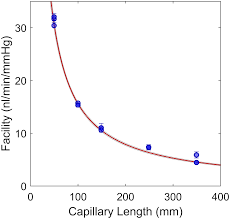The image above represents average outflow rate.

To compute for average outflow rate, three essential parameters are needed and these parameters are Average inflow rate (I), Change in storage during routing time (Δs) and Routing Time (Δt).

The formula for calculating average outflow rate:

O = I – Δs / Δt

Where:

O = Average Outflow Rate
I = Average Inflow Rate
Δs = Change in Storage during Routing Time
Δt = Routing Time

Let’s solve an example;
Given that the average inflow rate is 5, the change in storage during routing time is 2 and routing time is 10. Find the average outflow rate?

This implies that;

I = Average Inflow Rate = 5
Δs = Change in Storage during Routing Time = 2
Δt = Routing Time = 10

O = I – Δs / Δt
O = 5 – 2 / 10
O = 5 – 0.2
O = 4.8

Therefore, the average outflow rate is 4.8.

Calculating the Average Inflow Rate when the Average Outflow Rate, the Change in Storage during Routing Time and the Routing Time is Given.

I = O + Δs / Δt

Where;

I = Average Inflow Rate
O = Average Outflow Rate
Δs = Change in Storage during Routing Time
Δt = Routing Time

Let’s solve an example;
Find the Average Inflow Rate when the average outflow rate is 40, change in storage during routing time is 10 and the routing time is 5.

This implies that;

I = Average Inflow Rate = 40
Δs = Change in Storage during Routing Time = 10
Δt = Routing Time = 5

I = O + Δs / Δt
I = 40 + 105
I = 40 – 2
I = 38

Therefore, the average inflow rate is 38.

## How to Calculate and Solve for Average Inflow Rate | Hydrology II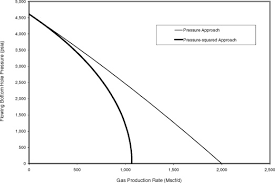The image above represents average inflow rate.

To compute for average inflow rate, three essential parameters are needed and these parameters are Average outflow rate (O), Change in storage during routing time (Δs) and Routing Time (Δt).

The formula for calculating average inflow rate:

I = O + Δs / Δt

Where:

I = Average Inflow Rate
O = Average Outflow Rate
Δs = Change in Storage during Routing Time
Δt = Routing Time

Let’s solve an example;
Find the Average Inflow Rate when the average outflow rate is 10, the change in storage during routing time is 5 and the routing time is 12.

This implies that;

O = Average Outflow Rate = 10
Δs = Change in Storage during Routing Time = 5
Δt = Routing Time = 12

I = O + Δs / Δt
I = 10 + 5 / 12
I = 10 + 0.416
I = 10.416

Therefore, the average inflow rate is 10.416.

Calculating the Average Outflow Rate when the Average Inflow Rate, the Change in Storage during Routing Time and the Routing Time is Given.

O = I – Δs / Δt

Where;

O = Average Outflow Rate
I = Average Inflow Rate
Δs = Change in Storage during Routing Time
Δt = Routing Time

Let’s solve an example;
Find the Average Outflow Rate when the average inflow rate is 50, change in storage during routing time is 20 and the routing time is 10.

This implies that;

I = Average Inflow Rate = 50
Δs = Change in Storage during Routing Time =20
Δt = Routing Time = 10

O = I – Δs / Δt
O = 50 – 20 / 10
O = 50 – 2
O = 48

Therefore, the average outflow rate is 48.

## How to Calculate and Solve for Unit Hydrograph | Hydrology II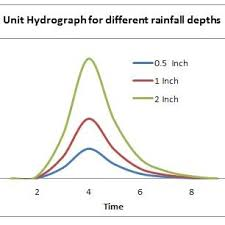The image above represents unit hydrograph.

To compute for unit hydrograph, two essential parameters are needed and these parameters are Direct run off (DRO) and Effective depth (ED).

The formula for calculating unit hydrograph:

UHG = DRO / ED

Where:

UHG = Unit Hydrograph
DRO = Direct Run Off
ED = Effective Depth

Let’s solve an example;
Find the unit hydrograph when the direct run off is 11 and the effective depth is 13.

This implies that:

DRO = Direct Run Off = 11
ED = Effective Depth = 13

UHG = DRO / ED
UHG = 11 / 13
UHG = 0.846

Therefore, the unit hydrograph is 0.846.

Calculating the Direct Run Off when the Unit Hydrograph and the Effective Depth is Given.

DRO = UHG x ED

Where;

DRO = Direct Run Off
UHG = Unit Hydrograph
ED = Effective Depth

Let’s solve an example;
Find the direct run off when the unit hydrograph is 21 and the effective depth is 10.

This implies that;

UHG = Unit Hydrograph = 21
ED = Effective Depth = 10

DRO = UHG x ED
DRO = 21 x 10
DRO = 210

Therefore, the direct run off is 210.

## How to Calculate and Solve for Annual Worth | Gradient Series | Economic Equivalence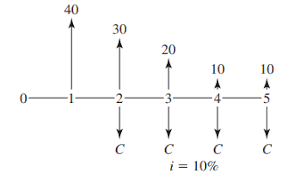The image above represents annual worth.

To compute for annual worth, three essential parameters are needed and these parameters are Gradient Amount (G), Interest Rate (i) and Number of Years (N).

The formula for calculating annual worth:

A = G[((1 + i)N) – iN – 1] / [((1 + i)N) – 1]

Where:

A = Annual Amount or Worth (Conversion Factor)
i = Interest Rate
N = Number of Years

Let’s solve an example;
Find the annual worth when the gradient amount is 11, the interest rate is 0.2 and the number of years is 12.

This implies that;

G = Gradient amount = 11
i = Interest Rate = 0.2
N = Number of Years = 12

A = G[((1 + i)N) – iN – 1] / [((1 + i)N) – 1]
A = 11[((1 + 0.2)12) – 0.2(12) – 1] / [((1 + 0.2)12) – 1]
A = 11[((1.2)12) – 2.40 – 1] / [((1.2)12) – 1]
A = 11[8.916 – 2.40 – 1] / [8.916 – 1]
A = 11[5.516] / [7.916]
A = 11 x 0.696
A = 7.66

Therefore, the annual worth is ₦7.66.

## How to Calculate and Solve for Present Worth | Geometric Gradient | Economic Equivalence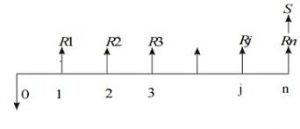The image above represents present worth.

To compute for present worth, four essential parameters are needed and these parameters are First Payment (A1), Interest Rate (i), Number of Year(s) (N) and Percentage change in payment (g).

The formula for calculating present worth:

P = A₁[1 – ((1 + g)N)((1 + i)-N)] / [1 – g]

Where:

P = Present Worth or Amount
A₁ = First Payment
i = Interest Rate
g = Percentage change in payment
N = Number of Years

Let’s solve an example;
Find the present worth when the first payment is 10, the interest rate is 0.2, the percentage change in payment is 20 and the number of years is 5.

This implies that;

A₁ = First Payment = 10
i = Interest Rate = 0.2
g = Percentage change in payment = 20
N = Number of Years = 5

P = A₁[1 – ((1 + g)N)((1 + i)-N)] / [1 – g]
P = 10[1 – ((1 + 20)5)((1 + i)-(5))] / [1 – 20]
P = 10[1 – ((21)5)((1.2)-5)] / [-19]
P = 10[1 – (4084101)(0.401)] / [-19]
P = 10[1 – 1641308.59] / [-19]
P = 10[-1641307.59] / [-19]
P = 10 x 86384.610
P = 863846.10

Therefore, the present worth is ₦863846.10.

## How to Calculate and Solve for Present Worth | Geometric Gradient | Economic Equivalence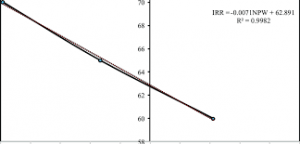The image above represents present worth.

To compute for present worth, three essential parameters are needed and these parameters are First Payment (A1), Interest Rate (i) and Number of Year(s) (N).

The formula for calculating present worth:

P = A₁[N / (1 + i)]

Where:

P = Present Worth or Amount
A₁ = First Payment
i = Interest Rate
N = Number of Years

Let’s solve an example;
Find the present worth when the first payment is 8, the interest rate is 0.3 and the number of years is 22.

This implies that;

A₁ = First Payment = 8
i = Interest Rate = 0.3
N = Number of Years = 22

P = A₁[N / (1 + i)]
P = 8[22 / (1 + 0.3)]
P = 8[22 / 1.3]
P = 8 x 16.92
P = 135.38

Therefore, the present worth is ₦135.38.

## How to Calculate and Solve for Present Worth | Gradient Series | Economic Equivalence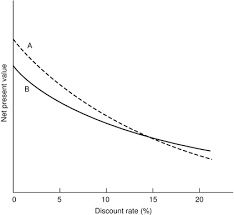The image above represents present worth.

To compute for present worth, three essential parameters are needed and these parameters are Gradient Amount (G), Interest Rate (i) and Number of Years (N).

The formula for calculating present worth:

P = G[((1 + i)N) – iN – 1] / [i²((1 + i)N)]

Where:

P = Present Amount or Worth
i = Interest Rate
N – Number of Years

Let’s solve an example;
Find the present worth when the gradient amount is 22, the interest rate is 0.2 and the number of years is 2.

This implies that;

G = Gradient Amount = 22
i = Interest Rate = 0.2
N – Number of Years = 2

P = G[((1 + i)N) – iN – 1] / [i²((1 + i)N)]
P = 22[((1 + 0.2)2) – 0.2(2) – 1] / [0.2²((1 + 0.2)2)]
P = 22[((1.2)2) – 0.4 – 1] / [0.0400000000000001((1.2)2)]
P = 22[1.44 – 0.4 – 1] / [0.04000000000000001 x 1.44]
P = 22[0.040000000000000036] / [0.05760000000000001]
P = 22 x 0.6944444444444449
P = 15.27

Therefore, the present worth is ₦15.27.

## How to Calculate and Solve for capital recovery | Equal Payment Series | Economic Equivalence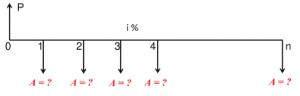The image above represents capital recovery.

To compute for capital recovery, three essential parameters are needed and these parameters are Present Amount or Worth (P), Interest Rate (i) and Number of Years (N).

The formula for calculating capital recovery:

A = P[i((1 + i)N)] / [((1 + i)N) – 1]

Where:

A = Annual Worth or Amount
P = Present Worth or Amount
i = Interest Rate
N = Number of Years

Let’s solve an example;
Find the annual worth when the present worth is 30, the interest rate is 0.2 and the number of years is 4.

This implies that;

P = Present Worth or Amount = 30
i = Interest Rate = 0.2
N = Number of Years = 4

A = P[i((1 + i)N)] / [((1 + i)N) – 1]
A = 30[0.2((1 + 0.2)4)] / [((1 + 0.2)4) – 1]
A = 30[0.2((1.2)4)] / [((1.2)4) – 1]
A = 30[0.2 x 2.0736] / [2.0736 – 1]
A = 30[0.41472] / [1.07359]
A = 30 x 0.386
A = 11.58

Therefore, the capital recovery is ₦11.58.

## How to Calculate and Solve for Present Worth | Equal Payment Series | Economic Equivalence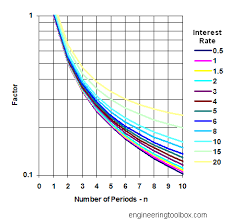The image above represent present worth.

To compute for present worth, three essential parameters are needed and these parameters are Annual Amount or Worth (A), Interest Rate (i) and Number of Years (N).

The formula for calculating present worth:

P = A[((1 + i)N) – 1] / [i((1 + i)N)]

Where:

P = Present Worth or Amount
A = Annual Worth or Amount
i = Interest Rate
N = Number of Years

Let’s solve an example;
Given that the annual worth is 29, the interest rate is 0.2 and the number of years is 10. Find the present worth?

This implies that;

A = Annual Worth or Amount = 29
i = Interest Rate = 0.2
N = Number of Years = 10

P = A[((1 + i)N) – 1] / [i((1 + i)N)]
P = 29[((1 + 0.2)10) – 1] / [0.2((1 + 0.2)10)]
P = 29[((1.2)10) – 1] / [0.2((1.2)10)]
P = 29[6.191 – 1] / [0.2 x 6.191]
P = 29[5.191] / [1.238]
P = 29 x 4.19
P = 121.58

Therefore, the present worth is ₦121.58.